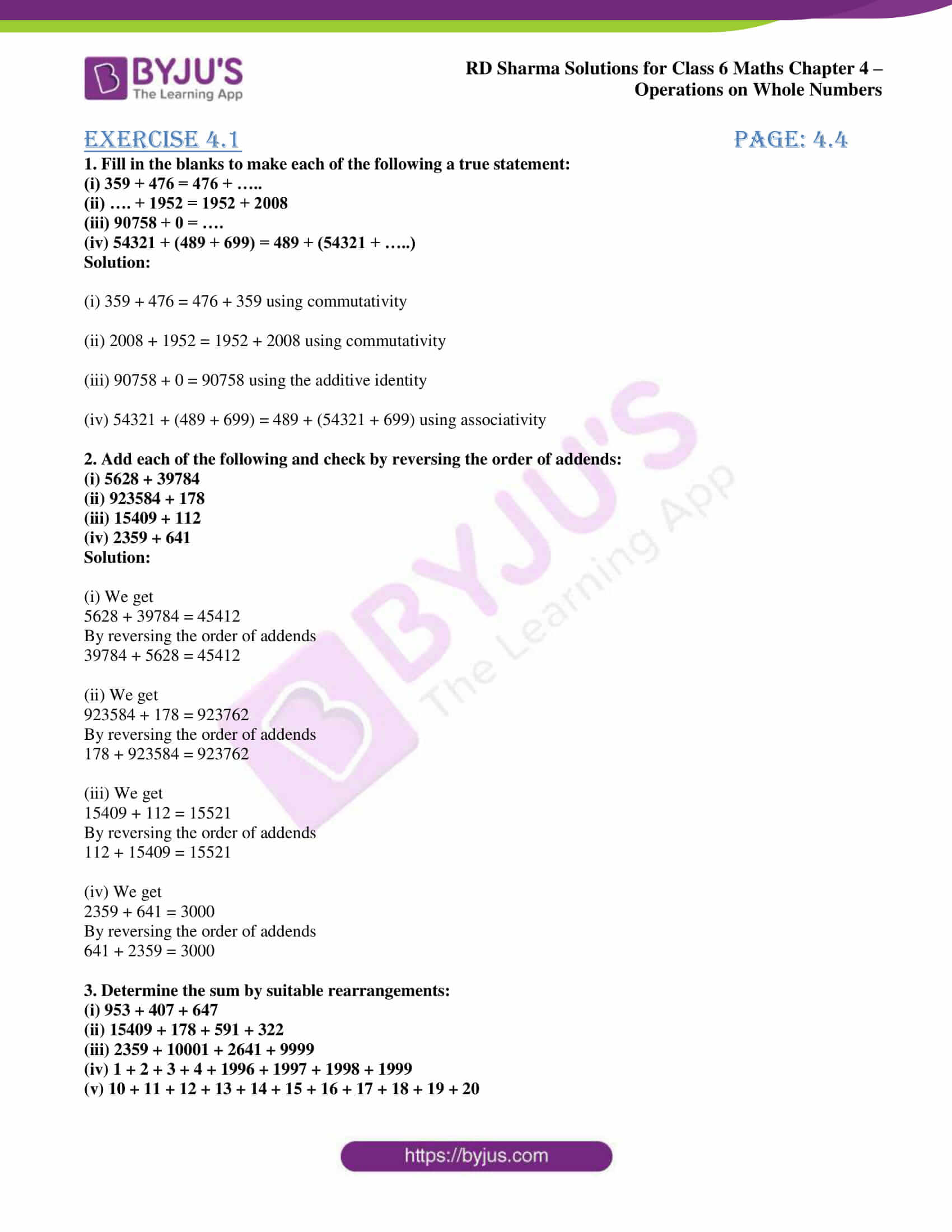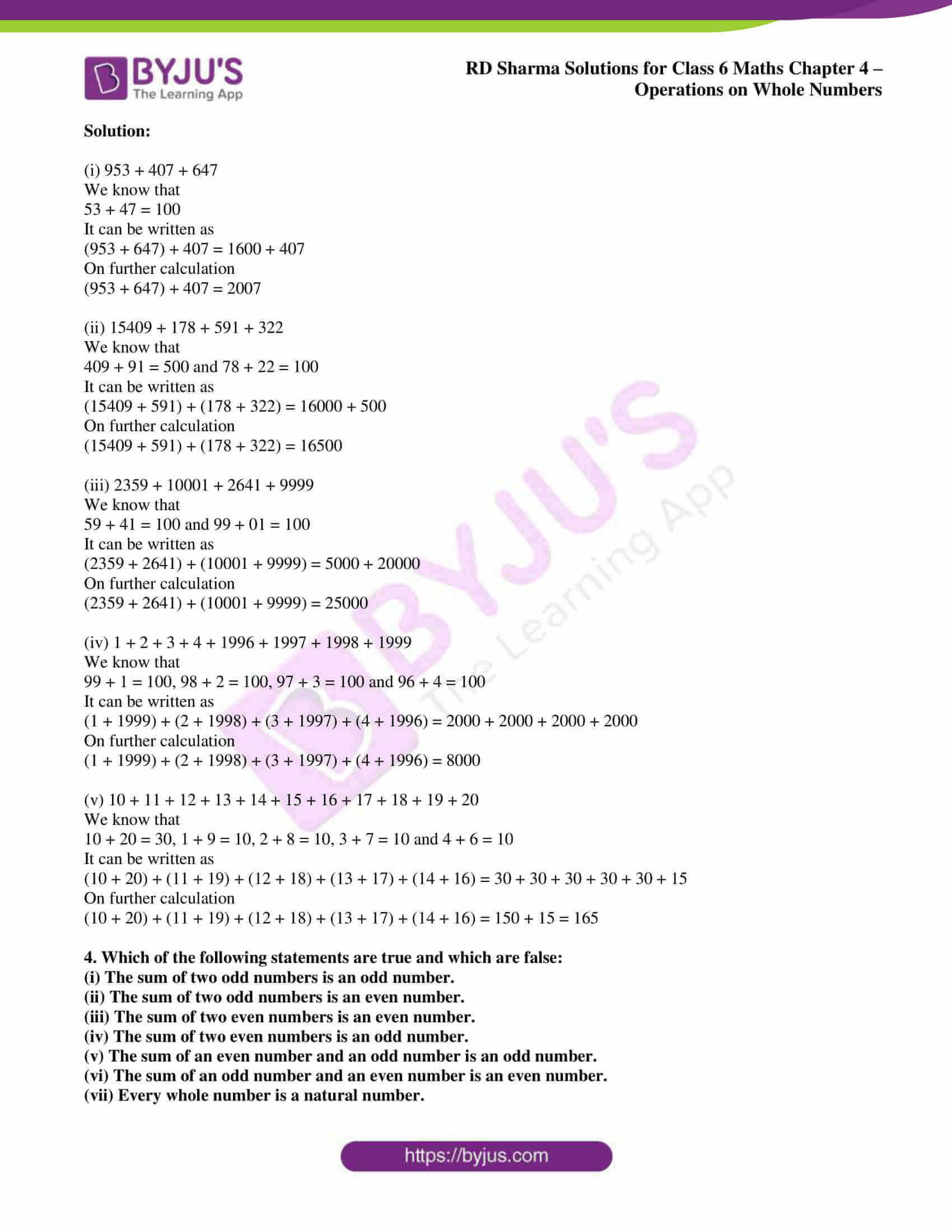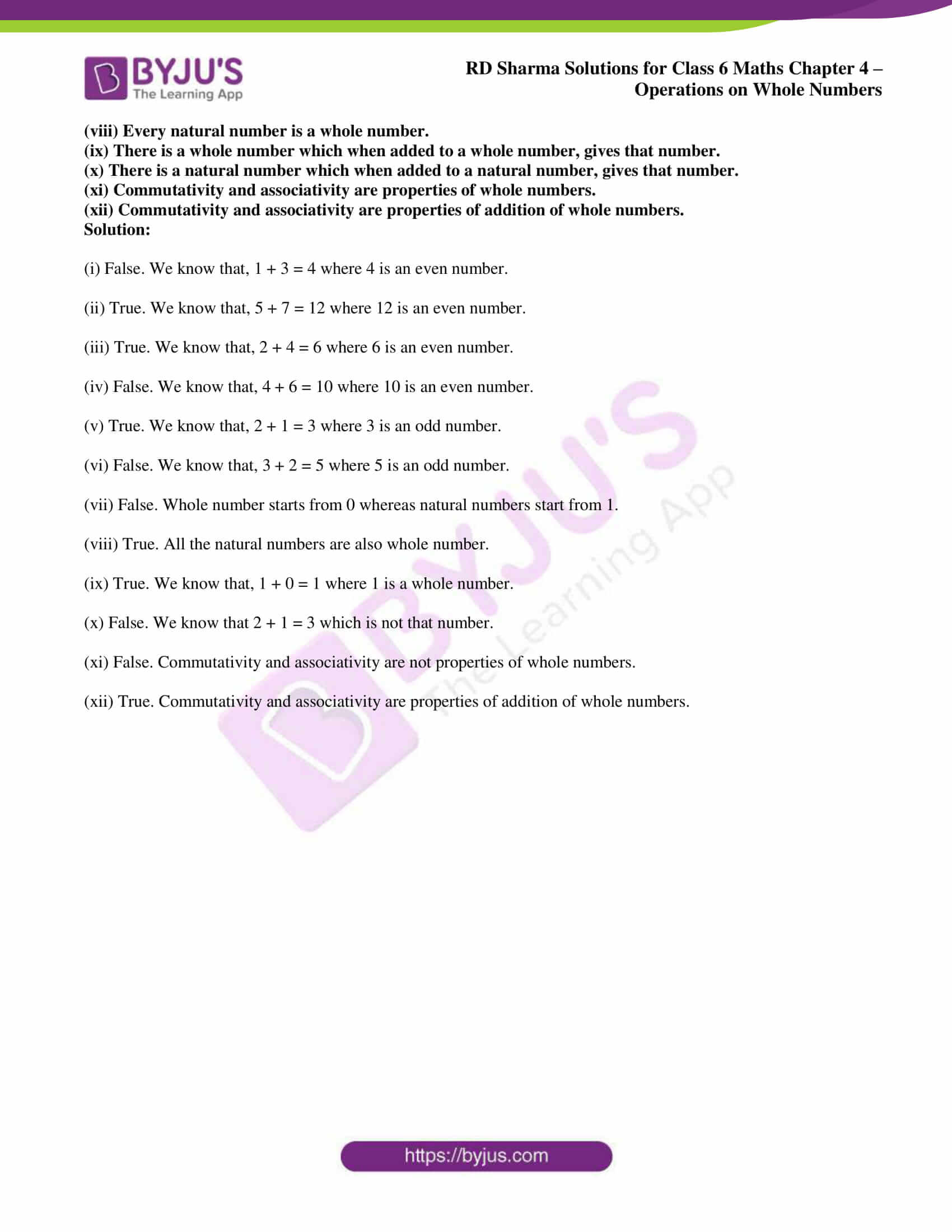# RD Sharma Solutions for Class 6 Maths Chapter 4: Operations on Whole Numbers Exercise 4.1

## RD Sharma Solutions for Class 6 Maths Exercise 4.1 PDF

The primary aim of creating solutions is to improve concept-based knowledge among students, which is essential for the exam. The solutions are well structured in a comprehensive manner based on the understanding capacity of the students. The subject experts prepare solutions after conducting wide research on each concept. The problems from RD Sharma textbook are solved based on the weightage in the exam. PDF of solutions can be downloaded and can be used while solving the exercise wise problems. RD Sharma Solutions for Class 6 Maths Chapter 4 Operations on Whole Numbers Exercise 4.1 are provided here.

## RD Sharma Solutions for Class 6 Maths Chapter 4: Operations on Whole Numbers Exercise 4.1 Download PDF## Access answers to Maths RD Sharma Solutions for Class 6 Chapter 4: Operations on Whole Numbers Exercise 4.1

1. Fill in the blanks to make each of the following a true statement:

(i) 359 + 476 = 476 + …..

(ii) …. + 1952 = 1952 + 2008

(iii) 90758 + 0 = ….

(iv) 54321 + (489 + 699) = 489 + (54321 + …..)

Solution:

(i) 359 + 476 = 476 + 359 using commutativity

(ii) 2008 + 1952 = 1952 + 2008 using commutativity

(iii) 90758 + 0 = 90758 using the additive identity

(iv) 54321 + (489 + 699) = 489 + (54321 + 699) using associativity

2. Add each of the following and check by reversing the order of addends:

(i) 5628 + 39784

(ii) 923584 + 178

(iii) 15409 + 112

(iv) 2359 + 641

Solution:

(i) We get

5628 + 39784 = 45412

By reversing the order of addends

39784 + 5628 = 45412

(ii) We get

923584 + 178 = 923762

By reversing the order of addends

178 + 923584 = 923762

(iii) We get

15409 + 112 = 15521

By reversing the order of addends

112 + 15409 = 15521

(iv) We get

2359 + 641 = 3000

By reversing the order of addends

641 + 2359 = 3000

3. Determine the sum by suitable rearrangements:

(i) 953 + 407 + 647

(ii) 15409 + 178 + 591 + 322

(iii) 2359 + 10001 + 2641 + 9999

(iv) 1 + 2 + 3 + 4 + 1996 + 1997 + 1998 + 1999

(v) 10 + 11 + 12 + 13 + 14 + 15 + 16 + 17 + 18 + 19 + 20

Solution:

(i) 953 + 407 + 647

We know that

53 + 47 = 100

It can be written as

(953 + 647) + 407 = 1600 + 407

On further calculation

(953 + 647) + 407 = 2007

(ii) 15409 + 178 + 591 + 322

We know that

409 + 91 = 500 and 78 + 22 = 100

It can be written as

(15409 + 591) + (178 + 322) = 16000 + 500

On further calculation

(15409 + 591) + (178 + 322) = 16500

(iii) 2359 + 10001 + 2641 + 9999

We know that

59 + 41 = 100 and 99 + 01 = 100

It can be written as

(2359 + 2641) + (10001 + 9999) = 5000 + 20000

On further calculation

(2359 + 2641) + (10001 + 9999) = 25000

(iv) 1 + 2 + 3 + 4 + 1996 + 1997 + 1998 + 1999

We know that

99 + 1 = 100, 98 + 2 = 100, 97 + 3 = 100 and 96 + 4 = 100

It can be written as

(1 + 1999) + (2 + 1998) + (3 + 1997) + (4 + 1996) = 2000 + 2000 + 2000 + 2000

On further calculation

(1 + 1999) + (2 + 1998) + (3 + 1997) + (4 + 1996) = 8000

(v) 10 + 11 + 12 + 13 + 14 + 15 + 16 + 17 + 18 + 19 + 20

We know that

10 + 20 = 30, 1 + 9 = 10, 2 + 8 = 10, 3 + 7 = 10 and 4 + 6 = 10

It can be written as

(10 + 20) + (11 + 19) + (12 + 18) + (13 + 17) + (14 + 16) = 30 + 30 + 30 + 30 + 30 + 15

On further calculation

(10 + 20) + (11 + 19) + (12 + 18) + (13 + 17) + (14 + 16) = 150 + 15 = 165

4. Which of the following statements are true and which are false:

(i) The sum of two odd numbers is an odd number.

(ii) The sum of two odd numbers is an even number.

(iii) The sum of two even numbers is an even number.

(iv) The sum of two even numbers is an odd number.

(v) The sum of an even number and an odd number is an odd number.

(vi) The sum of an odd number and an even number is an even number.

(vii) Every whole number is a natural number.

(viii) Every natural number is a whole number.

(ix) There is a whole number which when added to a whole number, gives that number.

(x) There is a natural number which when added to a natural number, gives that number.

(xi) Commutativity and associativity are properties of whole numbers.

(xii) Commutativity and associativity are properties of addition of whole numbers.

Solution:

(i) False. We know that, 1 + 3 = 4 where 4 is an even number.

(ii) True. We know that, 5 + 7 = 12 where 12 is an even number.

(iii) True. We know that, 2 + 4 = 6 where 6 is an even number.

(iv) False. We know that, 4 + 6 = 10 where 10 is an even number.

(v) True. We know that, 2 + 1 = 3 where 3 is an odd number.

(vi) False. We know that, 3 + 2 = 5 where 5 is an odd number.

(vii) False. Whole number starts from 0 whereas natural numbers start from 1.

(viii) True. All the natural numbers are also whole number.

(ix) True. We know that, 1 + 0 = 1 where 1 is a whole number.

(x) False. We know that 2 + 1 = 3 which is not that number.

(xi) False. Commutativity and associativity are not properties of whole numbers.

(xii) True. Commutativity and associativity are properties of addition of whole numbers.

### RD Sharma Solutions for Class 6 Maths Chapter 4 – Operations on Whole Numbers Exercise 4.1

RD Sharma Solutions Class 6 Maths Chapter 4 Operations on Whole Numbers Exercise 4.1 contains problems which are solved based on closure property, commutativity and associativity of addition.

### Key features of RD Sharma Solutions for Class 6 Maths Chapter 4: Operations on Whole Numbers Exercise 4.1

• RD Sharma Solutions help students to revise the entire syllabus based on CBSE guidelines.
• The solutions contain explanations for each step in a simple language to improve conceptual knowledge among students.
• Students can download the PDF of solutions both chapter wise or exercise wise based on the requirement.
• Important formulas, methods and shortcut tips are highlighted which are important from the exam point of view.